Next: Mass and Angular Momentum Up: Magnetohydrodynamic Fluids Previous: Parker Model of Solar

# Interplanetary Magnetic Field

Let us now investigate how the solar wind and the interplanetary magnetic field affect one another.

The hot coronal plasma making up the solar wind possesses an extremely high electrical conductivity. In such a plasma, we expect the concept of frozen-in'' magnetic field-lines, discussed in Sect. 5.3, to be applicable. The continuous flow of coronal material into interplanetary space must, therefore, result in the transport of the solar magnetic field into the interplanetary region. If the Sun did not rotate, the resulting magnetic configuration would be very simple. The radial coronal expansion considered above (with the neglect of any magnetic forces) would produce magnetic field-lines extending radially outward from the Sun.

Of course, the Sun does rotate, with a (latitude dependent) period of about 25 days.Since the solar photosphere is an excellent electrical conductor, the magnetic field at the base of the corona is frozen into the rotating frame of reference of the Sun. A magnetic field-line starting from a given location on the surface of the Sun is drawn out along the path followed by the element of the solar wind emanating from that location. As before, let us suppose that the coronal expansion is purely radial in a stationary frame of reference. Consider a spherical polar coordinate system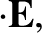which co-rotates with the Sun. Of course, the symmetry axis of the coordinate system is assumed to coincide with the axis of the Sun's rotation. In the rotating coordinate system, the velocity components of the solar wind are written(758)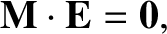(759)(760)

where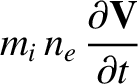is the angular velocity of solar rotation. The azimuthal velocity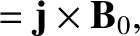is entirely due to the transformation to the rotating frame of reference. The stream-lines of the flow satisfy the differential equation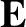(761)

at constant. The stream-lines are also magnetic field-lines, so Eq. (761) can also be regarded as the differential equation of a magnetic field-line. For radii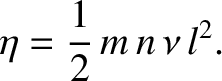greater than several times the critical radius,, the solar wind solution (757) predicts that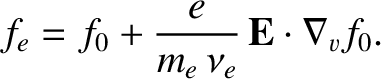is almost constant (see Fig. 18). Thus, forit is reasonable to write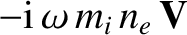, where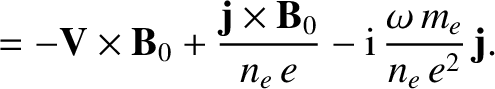is a constant. Equation (761) can then be integrated to give the equation of a magnetic field-line: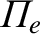(762)

where the field-line is assumed to pass through the point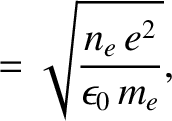. Maxwell's equation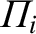, plus the assumption of a spherically symmetric magnetic field, easily yields the following expressions for the components of the interplanetary magnetic field: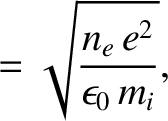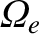(763)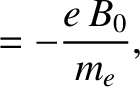(764)(765)

Figure 19 illustrates the interplanetary magnetic field close to the ecliptic plane. The magnetic field-lines of the Sun are drawn into spirals (Archemedian spirals, to be more exact) by the solar rotation. Transformation to a stationary frame of reference give the same magnetic field configuration, with the addition of an electric field(766)

The latter field arises because the radial plasma flow is no longer parallel to magnetic field-lines in the stationary frame.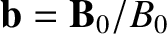The interplanetary magnetic field at 1 AU is observed to lie in the ecliptic plane, and is directed at an angle of approximatelyfrom the radial direction to the Sun. This is in basic agreement with the spiral configuration predicted above.

The analysis presented above is premised on the assumption that the interplanetary magnetic field is too weak to affect the coronal outflow, and is, therefore, passively convected by the solar wind. In fact, this is only likely to be the case if the interplanetary magnetic energy density,, is much less that the kinetic energy density,, of the solar wind. Rearrangement yields the condition(767)

where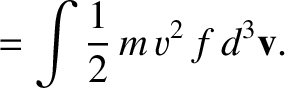is the Alfvén speed. It turns out thatat 1 AU. On the other hand,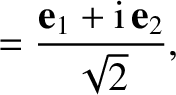close to the base of the corona. In fact, the solar wind becomes super-Alfvénic at a radius, denoted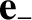, which is typically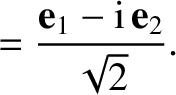, or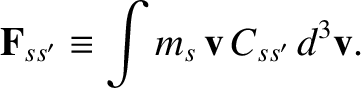of an astronomical unit. We conclude that the previous analysis is only valid well outside the Alfvén radius: i.e., in the region.

Well inside the Alfvén radius (i.e., in the region), the solar wind is too weak to modify the structure of the solar magnetic field. In fact, in this region we expect the solar magnetic field to force the solar wind to co-rotate with the Sun. Note that flux-freezing is a two-way-street: if the energy density of the flow greatly exceeds that of the magnetic field then the magnetic field is passively convected by the flow, but if the energy density of the magnetic field greatly exceeds that of the flow then the flow is forced to conform to the magnetic field.

The above discussion leads us to the following rather crude picture of the interaction of the solar wind and the interplanetary magnetic field. We expect the interplanetary magnetic field to be simply the undistorted continuation of the Sun's magnetic field for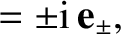. On the other hand, we expect the interplanetary field to be dragged out into a spiral pattern for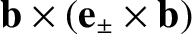. Furthermore, we expect the Sun's magnetic field to impart a non-zero azimuthal velocity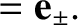to the solar wind. In the ecliptic plane, we expect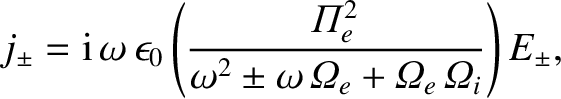(768)

for, and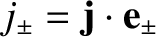(769)

for. This corresponds to co-rotation with the Sun inside the Alfvén radius, and outflow at constant angular velocity outside the Alfvén radius. We, therefore, expect the solar wind at 1 AU to possess a small azimuthal velocity component. This is indeed the case. In fact, the direction of the solar wind at 1 AU deviates from purely radial outflow by about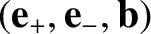.Next: Mass and Angular Momentum Up: Magnetohydrodynamic Fluids Previous: Parker Model of Solar
Richard Fitzpatrick 2011-03-31